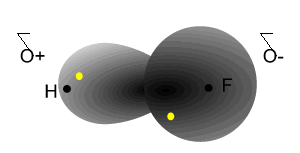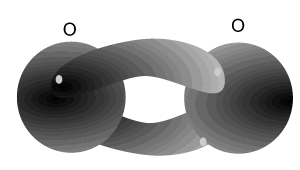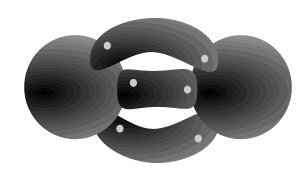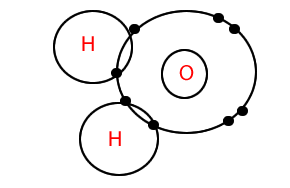Covalent bonding sharing of electrons amongst non-metals The simplified diagrams below only show electrons that are being shared. Click on the blue writing for more information Atoms that are one or more electrons short of a stable electron configuration can share electrons amongst themselves in a process called covalent bonding. Consider the two hydrogen atoms shown on the right. Each atom is one electron short of a stable configuration. They can combine to share one electron each and become stable by having two electrons each in their valence shells. The electrostatic attraction between the positive nucleus of each atom and the negative charged cloud created by the bonding electrons holds the the atoms tightly together to form molecules. The number of covalent bonds each atom forms depends on the number of electrons it requires to achieve a stable valence shell. Carbon forms molecules with other atoms. Carbon is four electrons short of a stable electron configuration so it will share four electrons with other non metals. Methane (CH4) is formed when carbon shares one electron with each of 4 hydrogen atoms. Each hydrogen has a stable two electrons while carbon has a stable 8 electrons in the valence shell. Water is a molecule formed between an oxygen and two hydrogen atoms. Ammonia is a molecule formed by nitrogen bonding to three hydrogens. A nitrogen atom needs three electrons to achieve a stable state, while each hydrogen needs one.Non-metal atoms have a high electronegativity (electron attracting power). When they react together what happens to their electrons? Are they given by one and taken by the other non-metal atom? NO! They share electrons. Sharing of electrons is known as covalent bonding. During covalent bonding, electrons from both non-metals travel between each other. Take the reaction between hydrogen and fluorine. Hydrogen needs one electron to complete its outer shell (2 electrons) while fluorine needs one electron to complete its outer shell (8 electrons). Both atoms attract an electron from each other.Notice how the electrons spend more time around the nucleus of fluorine. This will be discussed in more detail later.Atoms can even share electrons amongst themselves. The oxygen molecule is made up of two oxygen atoms covalently bonded(sharing electrons). Each atom needs two electrons to complete a stable (2,8) electron arrangement. So each atom shares two electrons with the other. When atoms share 4 electrons (2 electrons each) with each other we call this form of covalent bonding a double bond. Nitrogen atoms can bond with each other to form a triple bond. A triple covalent bond comes about when both atoms share 6 electrons (3 each). Each nitrogen atom needs 3 electrons to achieve a stable (2,8) electron arrangement. So each atom shares three electrons with the other.Molecules can be represented as Lewis Dot Diagrams. Consider the water molecule, shown on the right, it is represented as a Lewis dot diagram. To draw such a diagram follow two simple steps. 1) Draw each atom showing its valence electrons. Pair up the valence electrons but make sure you leave each bonding electron free. The number of bonding electrons is the number needed to reach a stable state. In this case oxygen has 6 valence electrons and needs two more electrons to become stable. Two electrons remain free on the oxygen but the others are paired. 2) Bring together the atoms so that the valence shells overlap. Sometimes the molecucle is represented with lines. To achieve this, replace each pair of electrons with a line. Look at the following molecules. N2 CH4The electrostatic attraction between the negative electrons being shared and the positive nuclei of each atom is very strong indeed. This electrostatic force holds the atoms strongly in the molecule. This force is so strong that it overcomes the repulsion between the positive nuclei of the atoms. The shared electrons are known as bonding electrons while the valence electrons that are not shared are known as non-bonding pairs or lone pairs. Continue How are the atoms held together in a covalent bond? attraction between each pair of bonding electrons repulsion between the nuclei of each atom attraction between the nuclei of each atom involved in the bond and the bonding electrons What determines the number of electrons each atom shares? number of electrons in the first energy level number of neutrons in the nucleus. number of electrons needed to achieve a stable state number of protons in the last energy level What type of atoms share electrons? metal atoms non-metal atoms Draw Lewis dot diagrams of the following molecules. SO3 NH3 CF4 CHF3 O2 Draw line diagrams of the molecules above and label the bonding and non-bonding electrons.